### IMO Shortlist 2012 problem A4

Kvaliteta:
Avg: 0,0
Težina:
Avg: 7,0
Let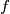$f$ and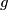$g$ be two nonzero polynomials with integer coefficients and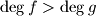$\deg f>\deg g$. Suppose that for infinitely many primes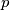$p$ the polynomial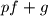$pf+g$ has a rational root. Prove that$f$ has a rational root.
Izvor: Međunarodna matematička olimpijada, shortlist 2012